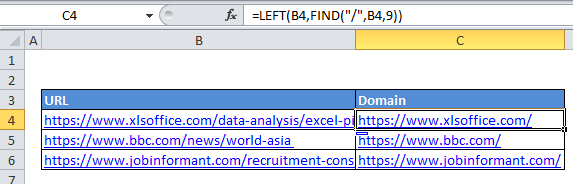# How to extract domain name from URL in Excel

If you want to extract the domain name from a complete URL, you can do so with a formula that uses  the LEFT and FIND functions. See example: In the formula below, url is the the URL you are working with.

## Formula

`=LEFT(url,FIND("/",url,9))`## Explanation

In the example, we are using this formula:

`=LEFT(B4,FIND("/",B4,9))`

### Here’s how the formula works:

B4 contains the URL: “

Also See:   Remove first character in a cell in Excel

At the core, this formula is extracting characters from the URL, starting from the left, and using the FIND function to figure out how many characters to extract.

First, FIND locates the “/” character in the URL, starting at the 9th character. This is the “clever” part of the formula. URLs begin with something called a “protocol” which looks like this:

Also See:   How to create email address from name in Excel

ftp://
sftp://
http://
https://

and so on. By starting at the 9th character, the protocol is skipped, and the FIND function will return the location of the 3rd instance of “/” (the first instance after the double slash in the protocol).

In this case, the third instance of “/” is the 21st character in the URL, so FIND returns the number 21.

Also See:   Extract word that begins with specific character in Excel

The LEFT function then extracts 21 characters from the URL, starting at the left. The result is the domain name with a trailing slash.

If you want to get the domain name without a trailing slash, just subtract the number 1 from the the result of FIND like so:

`=LEFT(B4,FIND("/",B4,9)-1)`